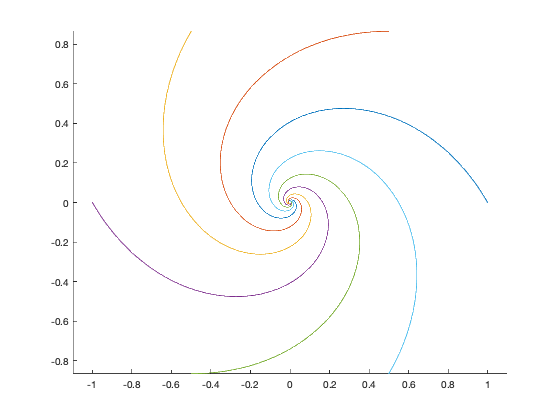# How to Write Animated PNG (APNG) Files from MATLAB

Today, I'm writing about how to create animated PNG (APNG) files, such as this one:I first wrote about APNG files last February. An APNG file is a valid PNG image file that uses file metadata in a clever way to compress and store the frames of a full animation. See the 07-Feb-2019 post for more details about this.

Just recently, I created a MATLAB utility for creating APNG files. It is available on the File Exchange and also on GitHub. This utility makes use of the program APNG Assember, which is available under separate license terms. The utility will automatically download this program for you the first time you use it.

To demonstrate its use, I will use it to create an animation of some pursuit curves that I first showed in my 14-Feb-2019 blog post. Here is the code for one version of the pursuit curves:

theta = 0:60:330;
P = cosd(theta) + 1i*sind(theta);
P = reshape(P,1,[]);
N = length(P);
d = 0.01;
for k = 1:1000
V = P(end,[(2:N) 1]) - P(end,:);
P(end+1,:) = P(end,:) + d*V;
end
hold on
for k = 1:N
plot(real(P(:,k)),imag(P(:,k)))
end
hold off
axis equalThis relatively simplistic way to compute the curves doesn't work so well for animating them, however, because the spacing of the computed points gets smaller and smaller as the points get closer to the center. The animation would appear to slow down.

So, I wrote a little code to resample the generated curve in approximately equal arc-length segments. Here's what that looks like:

step_distance = abs(diff(P(:,1),1));
cumulative_step_distance = [0; cumsum(step_distance,1)];
max_travel_distance = cumulative_step_distance(end);
num_resampled_steps = 10000;
x = linspace(0,max_travel_distance,num_resampled_steps);
Pr = interp1(cumulative_step_distance,P,x);


And here's the code to generate one frame of the animation at a time.

num_frames = 100;
q = round(linspace(1,size(Pr,1),num_frames));

fig = gcf;
fig.Position(3:4) = 300;

frames = zeros(0,0,'uint8');
for k = 1:length(q)
clf
hold on
for v = 1:N
plot(real(Pr(1:q(k),v)),imag(Pr(1:q(k),v)))
end
hold off
axis(limits)
axis equal
axis off
frame = print(fig,'-r150','-RGBImage');
frames = cat(4,frames,frame);
endHere's an extra wrinkle. Although APNG is widely supported by browsers today, there are plenty of programs around that display image files and that don't know about the extra animation metadata in APNG files. I want these viewers to show the last frame of the animation because that's the frame that includes the complete curves. So I will prepend that frame to the beginning, and then I'll tell the APNG utility class to skip the first frame for animations.

frames = cat(4,frame,frames);

% Create the APNG file.
w = animatedPNGWriter('animated-pursuit-curves.png');
w.FramesPerSecond = 20;
w.SkipFirstFrame = true;
for k = 1:size(frames,4)
end
finish(w);


Download and give animatedPNGWriter a try. If you find trouble with it, feel free to report an issue on its GitHub repository.

Published with MATLAB® R2019b

|